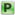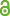Hauptmenü
• Autor
• Berkes, István
• Tichy, Robert
• TitelThe Kadec-Pelczynski theorem in $$L^p$$ , $$1 ≤ p 2$$
• Datei
• DOI10.1090/proc/12872
• Erschienen inProceedings of the American Mathematical Society
• Band144
• Erscheinungsjahr2016
• Heft5
• Seiten2053-2066
• LicenceCC BY
• ISSN1088-6826
• Zugriffsrechte• AbstractBy a classical result of Kadec and Pe lczynski (1962), every normalized weakly null sequence in $$L^p$$ , $$p > 2$$ contains a subsequence equivalent to the unit vector basis of $$l^2$$ or to the unit vector basis of $$l^p$$ . In this paper we investigate the case $$1 ≤ p < 2$$ and show that a necessary and sufficient condition for the first alternative in the Kadec-Pelczynski theorem is that the limit random measure μ of the sequence satisfies $$∫_{\mathbb{R}} x^2 dμ(x) ∈ L^{p/2}$$ .